Area of sector of circle

Chapter 12 Class 10 Areas related to Circles
Concept wise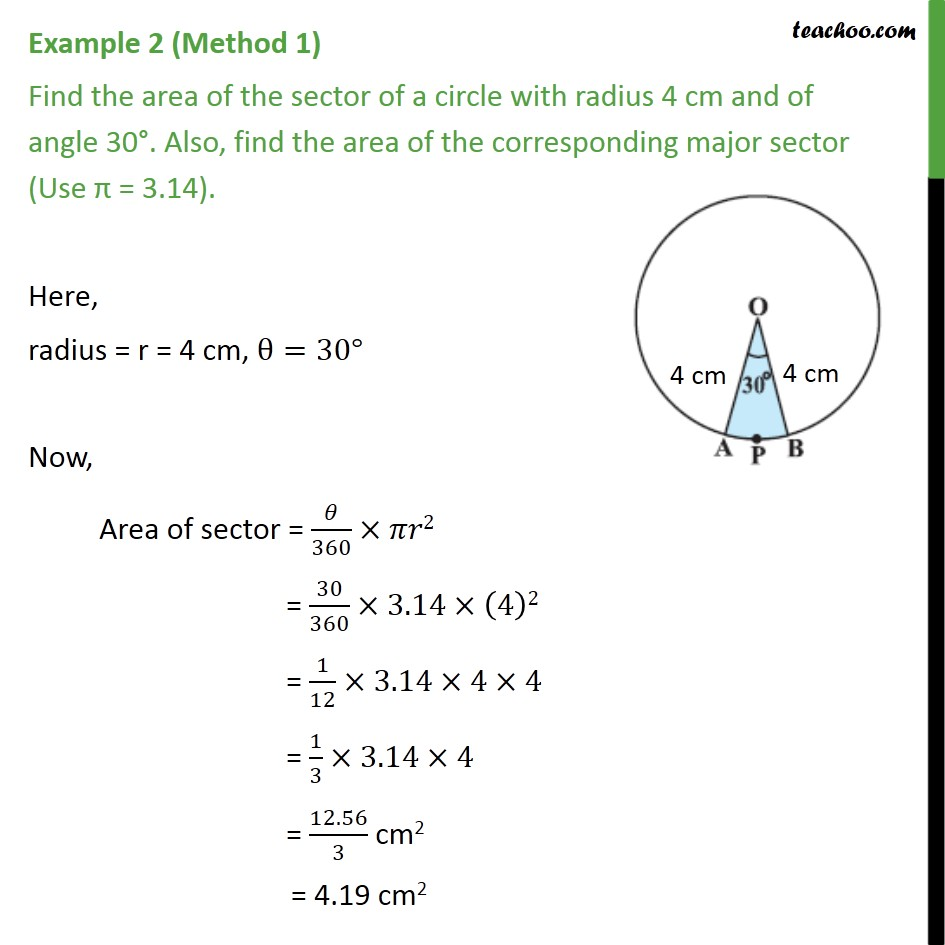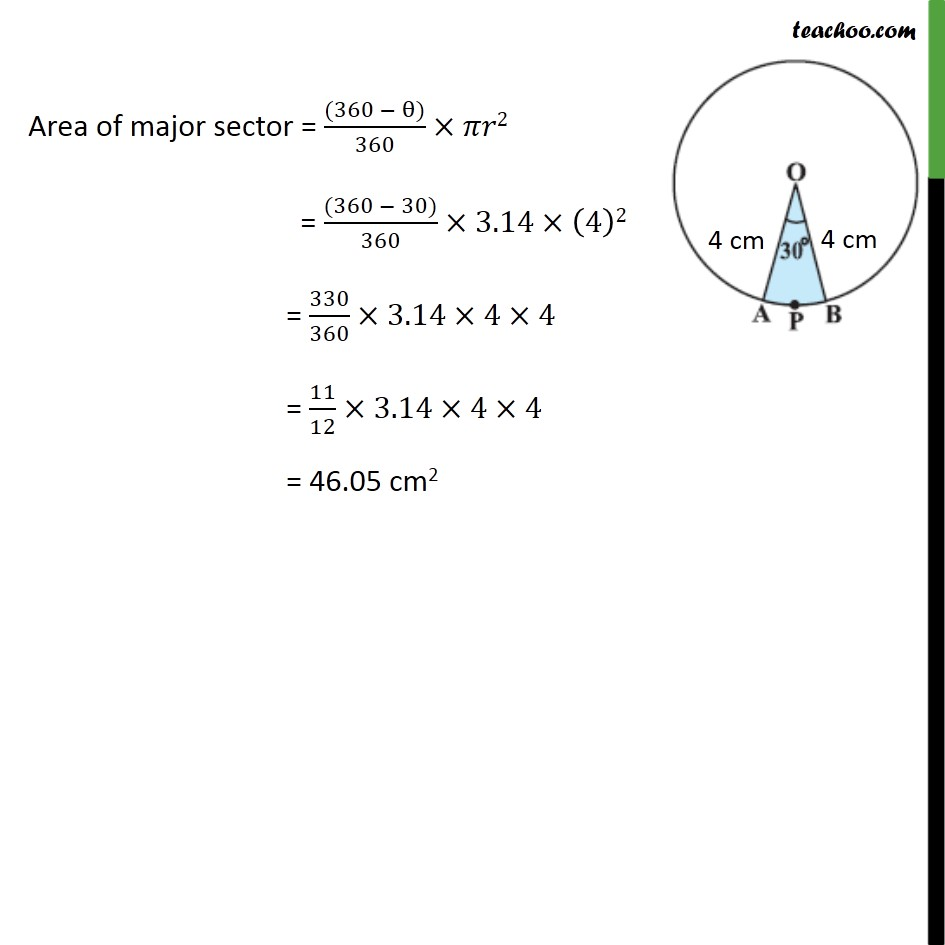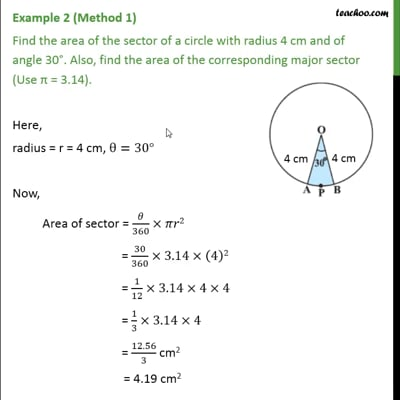This video is only available for Teachoo black users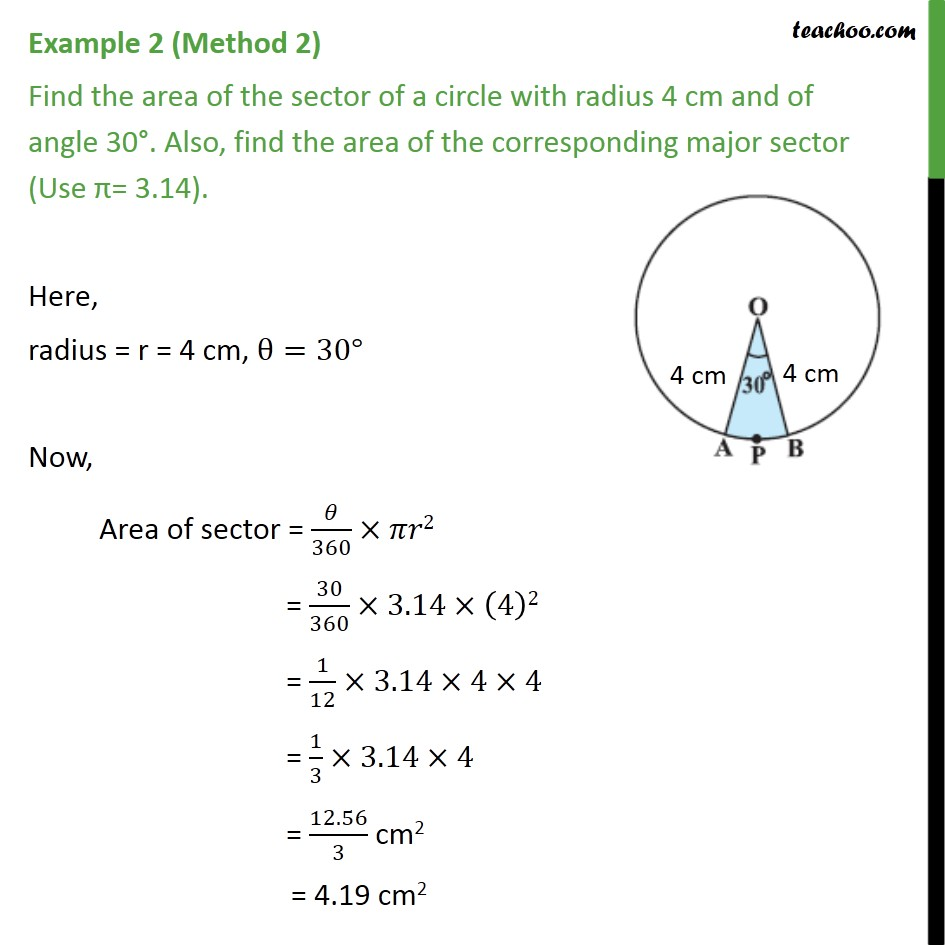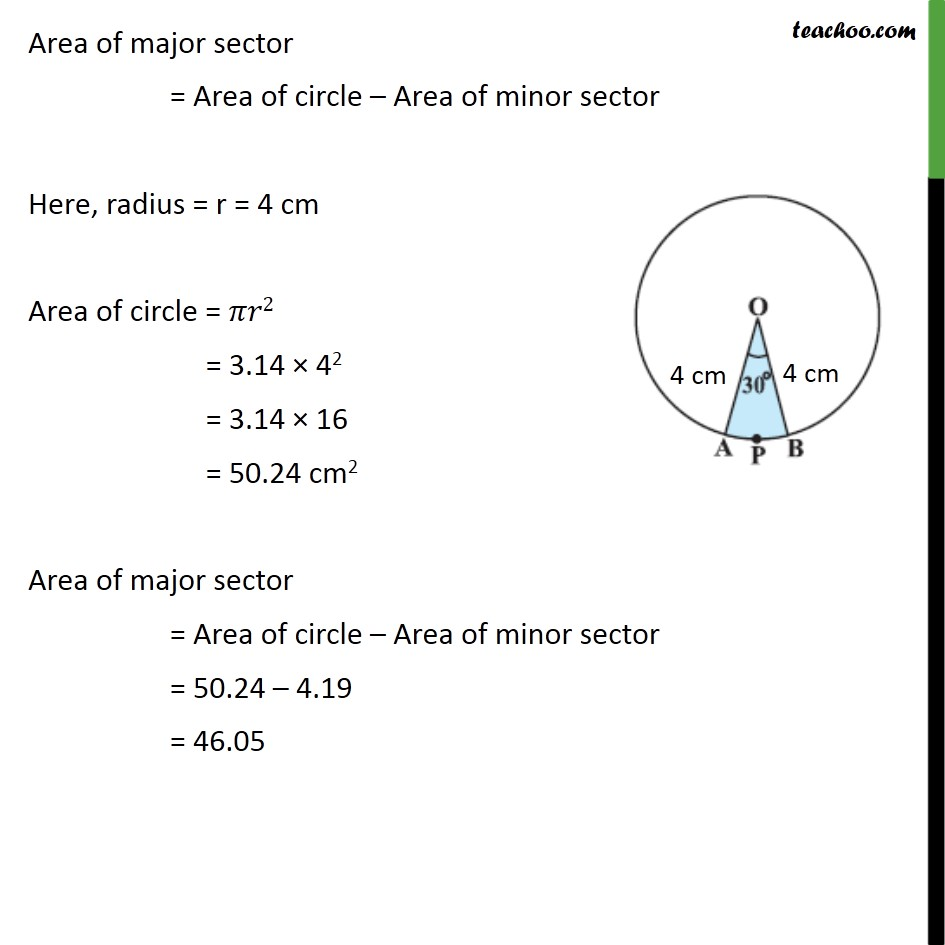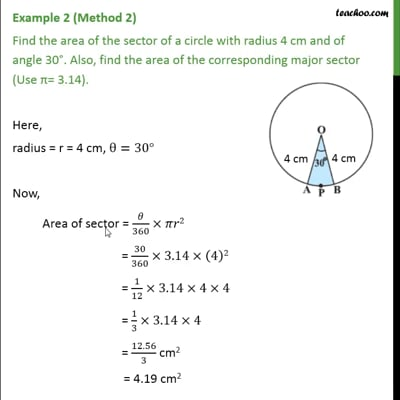This video is only available for Teachoo black users

Solve all your doubts with Teachoo Black (new monthly pack available now!)

### Transcript

Example 2 (Method 1) Find the area of the sector of a circle with radius 4 cm and of angle 30°. Also, find the area of the corresponding major sector (Use π = 3.14). Here, radius = r = 4 cm, θ=30° Now, Area of sector = 𝜃/360×𝜋𝑟2 = 30/360×3.14×(4)2 = 1/12×3.14×4×4 = 1/3×3.14×4 = 12.56/3 cm2 = 4.19 cm2 Area of major sector = ((360 − θ))/360×𝜋𝑟2 = ((360 − 30))/360×3.14×(4)2 = 330/360×3.14×4×4 = 11/12×3.14×4×4 = 46.05 cm2 Example 2 (Method 2) Find the area of the sector of a circle with radius 4 cm and of angle 30°. Also, find the area of the corresponding major sector (Use π= 3.14). Here, radius = r = 4 cm, θ=30° Now, Area of sector = 𝜃/360×𝜋𝑟2 = 30/360×3.14×(4)2 = 1/12×3.14×4×4 = 1/3×3.14×4 = 12.56/3 cm2 = 4.19 cm2 Area of major sector = Area of circle – Area of minor sector Here, radius = r = 4 cm Area of circle = 𝜋𝑟2 = 3.14 × 42 = 3.14 × 16 = 50.24 cm2 Area of major sector = Area of circle – Area of minor sector = 50.24 – 4.19 = 46.05